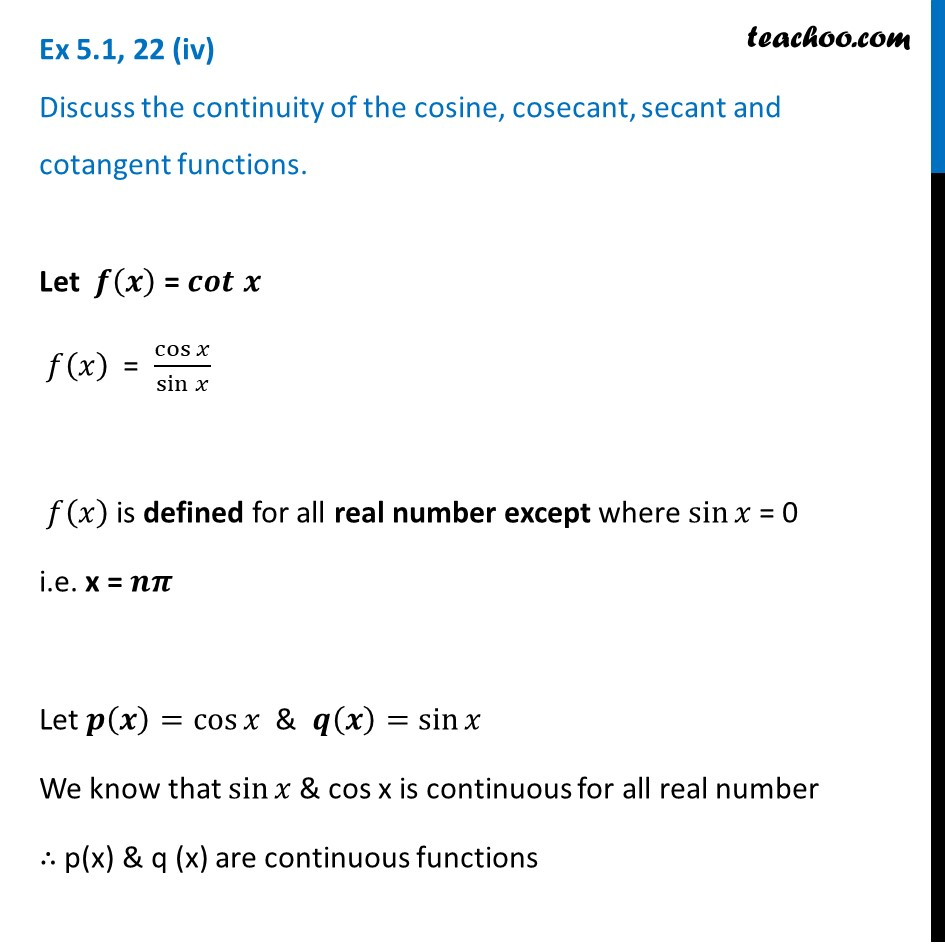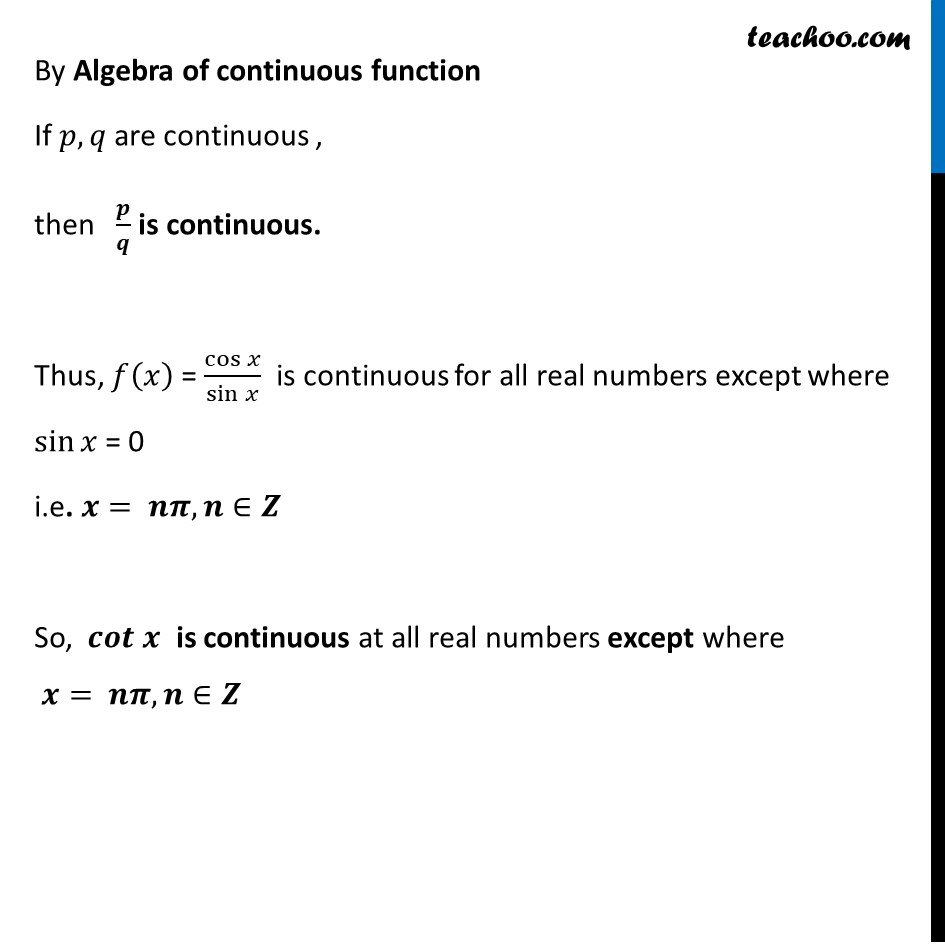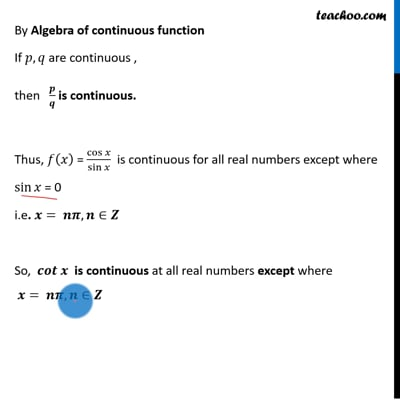Ex 5.1

Chapter 5 Class 12 Continuity and Differentiability
Serial order wiseThis video is only available for Teachoo black users

Introducing your new favourite teacher - Teachoo Black, at only ₹83 per month

### Transcript

Ex 5.1, 22 (iv) Discuss the continuity of cotangent functions.Let 𝒇(𝒙) = 𝒄𝒐𝒕 𝒙 𝑓(𝑥) = cos⁡𝑥/sin⁡𝑥 𝑓(𝑥) is defined for all real number except where sin⁡𝑥 = 0 i.e. x = 𝒏𝝅 Let 𝒑(𝒙)=cos⁡𝑥 & 𝒒(𝒙)=sin⁡𝑥 We know that sin⁡𝑥 & cos x is continuous for all real number ∴ p(x) & q (x) are continuous functions By Algebra of continuous function If 𝑝, 𝑞 are continuous , then 𝒑/𝒒 is continuous. Thus, 𝑓(𝑥) = cos⁡𝑥/sin⁡𝑥 is continuous for all real numbers except where sin⁡𝑥 = 0 i.e. 𝒙= 𝒏𝝅, 𝒏∈𝒁 So, 𝒄𝒐𝒕 𝒙 is continuous at all real numbers except where 𝒙= 𝒏𝝅, 𝒏∈𝒁